# Python OpenCV image area contour mark, which can be used to frame various small notes

2022-01-30 14:13:47

This article has participated in  「 Digging force Star Program 」 , Win a creative gift bag , Challenge creation incentive fund .

Python OpenCV 365 Day study plan , Go into the field of image with the eraser . This blog is the third in this series 54 piece .

Part 1 OpenCV The blog originally planned to complete a Identification bank card number Project , But in the process of writing, I found , Insufficient technical reserves , I can't in the following picture , Extract the card number area , There will be no subsequent identification , Once again realize that their technology is not up to standard , Continue to learn . can't finish , To achieve other learning projects .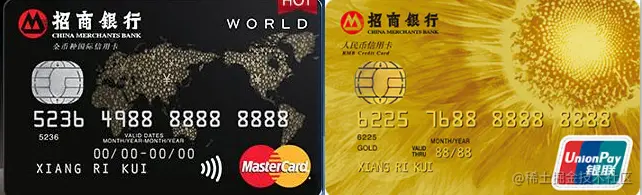## Contour recognition practice

Let's take a look at the final results , For a picture （ The foreground and background colors of the picture are quite different ）, Make contour marks .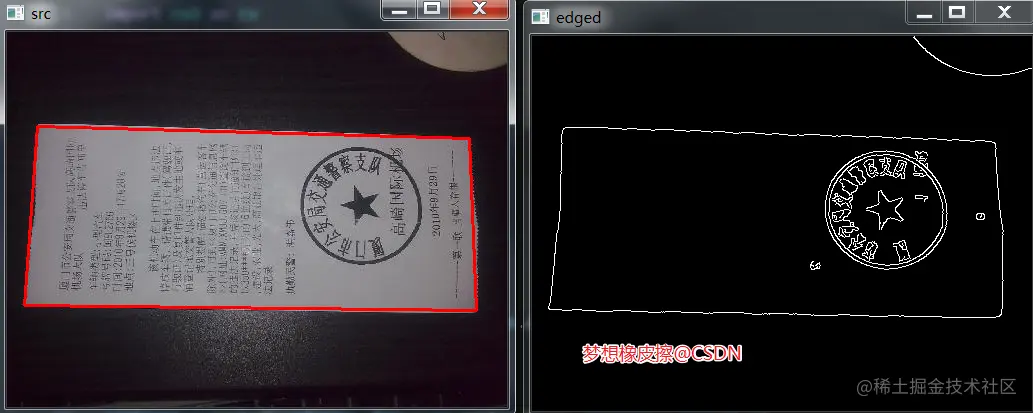### Basic image processing

``````import cv2 as cv

gray = cv.cvtColor(src, cv.COLOR_BGR2GRAY)
# cv.imshow("src", src)

gray = cv.GaussianBlur(gray, (5, 5), 0)
edges = cv.Canny(gray, 70, 210)

cv.imshow("edged", edges)
Copy code ``````

Convert to grayscale , Gaussian blur denoising ,Canny edge detection , These are the basic functions of image processing , The usage method has been studied above .

After running the above code , Get basic edge data .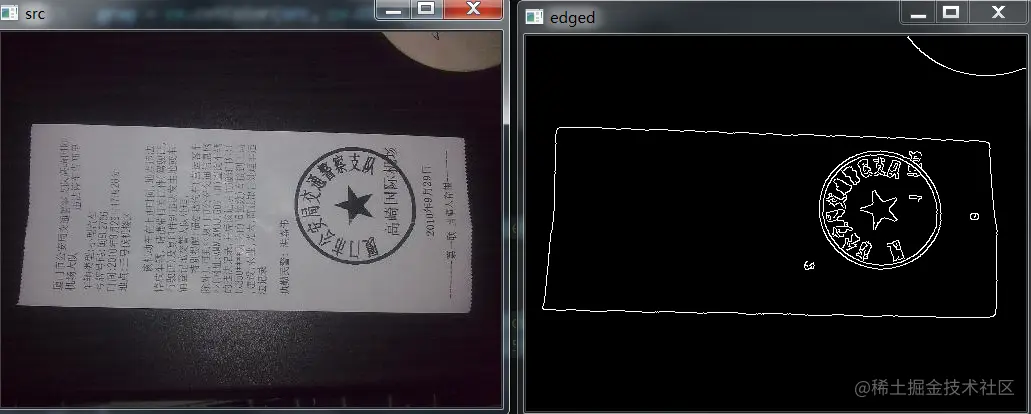### Contour detection

The following is the code to detect the specific position of the image contour ：

``````contours, hierarchy = cv.findContours(edges.copy(), cv.RETR_LIST, cv.CHAIN_APPROX_SIMPLE)
print(f" Number of contours ：{len(contours)}")
Copy code ``````

stay `cv.findContours(edges, cv.RETR_LIST, cv.CHAIN_APPROX_SIMPLE)` in , The second parameter uses `cv.RETR_LIST`, This parameter value indicates that all contours are detected , Do not establish hierarchical relationships , Mutual independence . If you only want to get contour edge information , Don't care if it's nested within another contour , Use this parameter value .

The third parameter uses `cv.CHAIN_APPROX_SIMPLE`, Indicates the horizontal direction of compression , vertical direction , Diagonal elements , Only the coordinates of the end point in this direction are reserved , For example, a rectangular outline only needs 4 Points to save profile information , This is also for the convenience of calculation later .

To observe the above , It can be found that the outermost edge area is the largest , So sort by area , Depending on other values , Get the contour with the largest area .

``````contours = sorted(contours, key = cv.contourArea, reverse = True)[:3]
Copy code ``````

Simply draw the outline , Get the image below .

``````cv.drawContours(src,contours,-1,(0,0,255),2)

Copy code ``````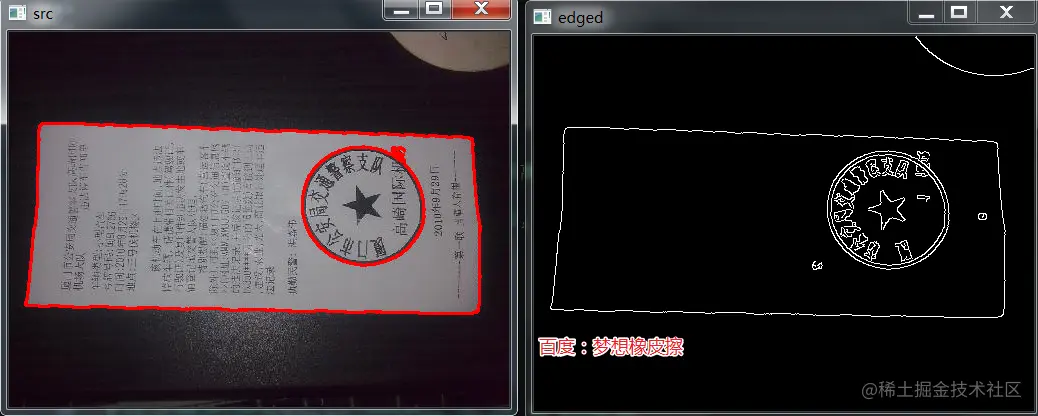### Traverse the outline , Calculate the contour approximation

Look at the code first ：

``````#  Traverse the outline
for c in contours:
#  Calculate the contour approximation
peri = cv.arcLength(c, True)
approx = cv.approxPolyDP(c, 0.02 * peri, True)
Copy code ``````

A new function `cv.arcLength`, The prototype of this function is as follows ：

``````retval = cv2.arcLength(curve, closed)
Copy code ``````

This function is used to calculate the perimeter of the contour .

Below `cv.approxPolyDP` The function prototype is as follows ：

``````approxCurve = cv2.approxPolyDP(curve, epsilon, closed[, approxCurve])
Copy code ``````

The function parameters are as follows ：

• `curve`： An outline of the source image ;
• `epsilon`： Distance value , Indicates how close the contour of a polygon is to the actual contour , The smaller the value. , The more accurate ;
• `closed`： Whether the contour is closed .

The most important parameter is `epsilon` Simple memory is ： The smaller the value , The more polygon corners you get , The closer to the actual contour , This parameter is an accuracy parameter .

The return value of this function is the corner of the contour approximate polygon .

### Draw the outline

Finally, judge , When the corner returned above is 4 When , Extract outline , The code is as follows ：

``````#  Traverse the outline
for c in contours:
#  Calculate the contour approximation
peri = cv.arcLength(c, True)
approx = cv.approxPolyDP(c, 0.02 * peri, True)

#  When it happens to be  4  At a corner , Get profile .
if len(approx) == 4:
screen_cnt = approx
break

#  Results show
cv.drawContours(src, [screen_cnt], -1, (0, 0, 255), 2)
Copy code ``````

Change pictures , Perform contour detection again , Pay attention to modifying the code of the approximate part of the contour .

``````#  Traverse the outline
for c in contours:
#  Calculate the contour approximation

approx = cv.approxPolyDP(c, 30, True)

if len(approx) == 4:
screen_cnt = approx
break
Copy code ``````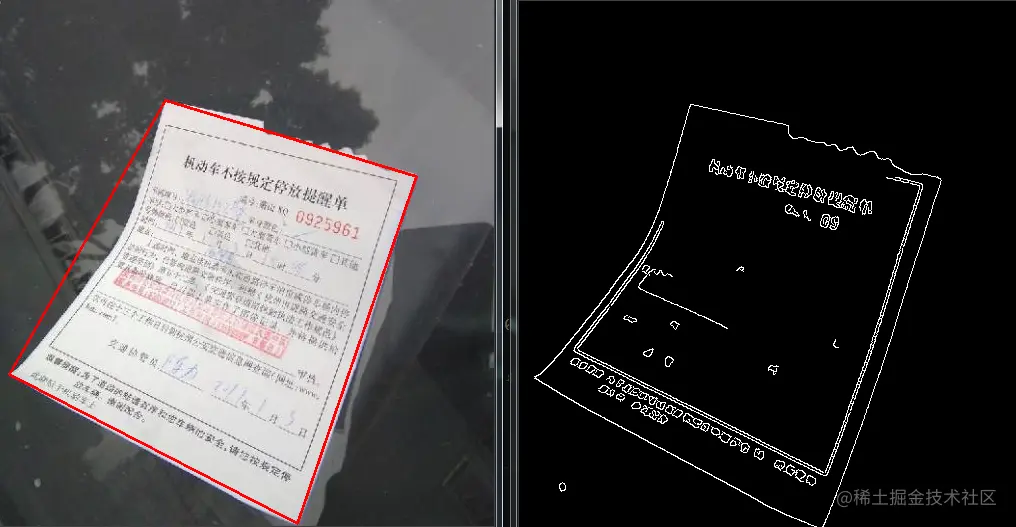## Eraser bars

I hope today's 1 Hours （ It doesn't seem to be enough ） You get something , I'll see you on our next blog ~